00 970 569 831 045 info@ajsrp.com

Application of Artificial Neural Networks Model for Forecasting Consumption of Electricity in Gezira State, Sudan (2006-2018)

Faculty of Science || University of Hafr Albatin || KSA

Abstract: This paper aimed applying models of artificial neural networks to electricity consumption data in the Gezira state, Sudan for the period (Jan 2006- May 2018), and predicting future values for the period (Jun 2018- Dec 2020) by train a recurrent neural network using Quasi-Newton Sampling and using online learning. The study relied on data from the national control center. After applying artificial neural networks, The Thiel coefficient is used to confirm the efficiency of the model, and the paper recommends the use of artificial neural networks to various time series data due to their strength and Accuracy. Keywords: Artificial Neural Networks, Thiel coefficient, electricity consumption.

تطبيقات الشبكات العصبية الاصطناعية للتنبؤ باستهلاك الكهرباء في ولاية الجزيرة، السودان (2006-2018)

ندى محمد أحمد الأمين

كلية العلوم || جامعة حفر الباطن || المملكة العربية السعودية

الملخص: هدفت هذه الورقة إلى تطبيق نماذج الشبكات العصبية الاصطناعية على بيانات استهلاك الكهرباء في ولاية الجزيرة, السودان للفترة (يناير 2006 – مايو 2018) والتنبؤ بالقيم المستقبلية للفترة (يونيو 2018 – ديسمبر 2020), وقد اعتمدت الدراسة على البيانات التي كان مصدرها مركز التحكم القومي وبعد تطبيق الشبكات العصبية الاصطناعية عليها توصلنا إلى نتائج فعالة تم فحص فعاليتها باستخدام معامل ثايل لتقييم دقة التنبؤات التي تم التوصل إليها, وقد أوصت الدراسة باستخدام الشبكات العصبية الاصطناعية لنمذجة مختلف السلاسل الزمنية نظراً لقوتها ودقتها. الكلمات المفتاحية: الشبكات العصبية الاصطناعية، معامل ثايل, استهلاك الكهرباء.

# 1.           Introduction:

Forecasting electricity consumption is one of the most important factors affecting the success of investment in different sectors, as electricity is the main energy source in Sudan and in order to make any decision in any economic area must provide the power operating energy commensurate with the quantity and cost with the expected consumption of Electric power. The importance of forecasting also comes from the possibility of determining the expected changes to consumption negatively and positively depending on the factors affecting it. The method of analysis of time series is preferred for prediction purposes in two cases, the first of which is when there are problems either in finding external factors affecting the phenomenon or problems in measuring the relationships that govern this behavior or both.

Second, when the primary objective of the prediction is to know the value of the phenomenon or the behavior of the phenomenon in the future only without the need to explain this behavior.

Neural network forecasting is more flexible than typical linear or polynomial approximations and is thus more precise. With neural networks an expert can discover and take into account non-linear connections and relationships between data and build a candidate model with high prediction strength

The most popular definition of artificial neural networks is a mathematical model that tries to simulate the structure and functionalities of biological neural networks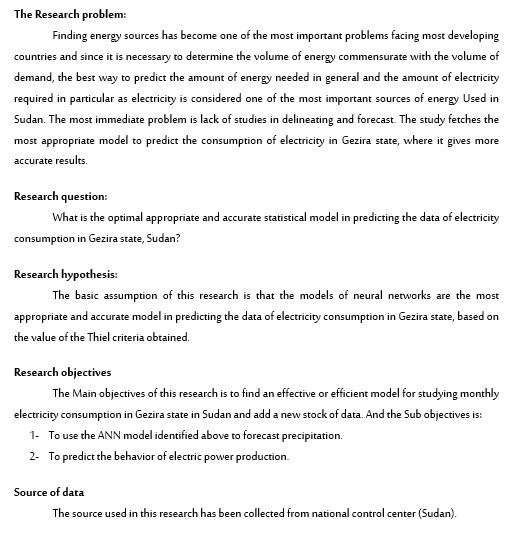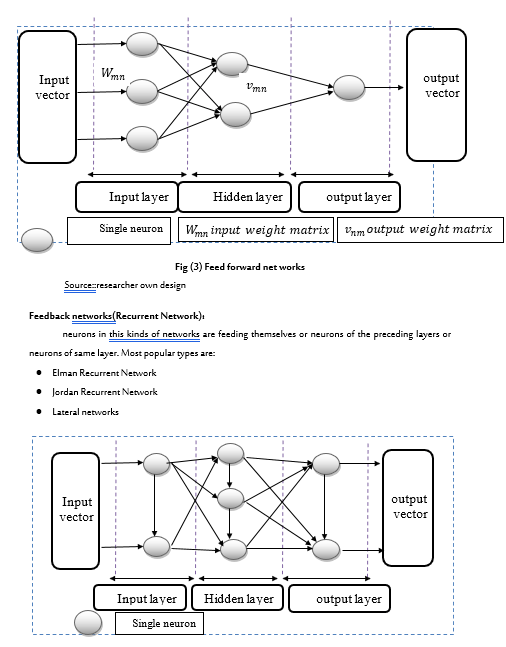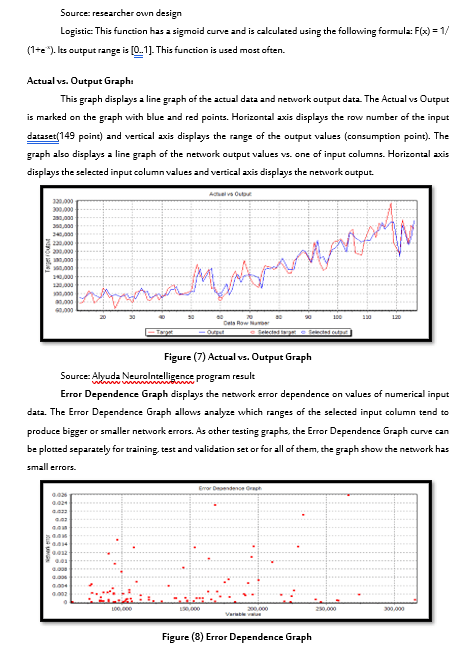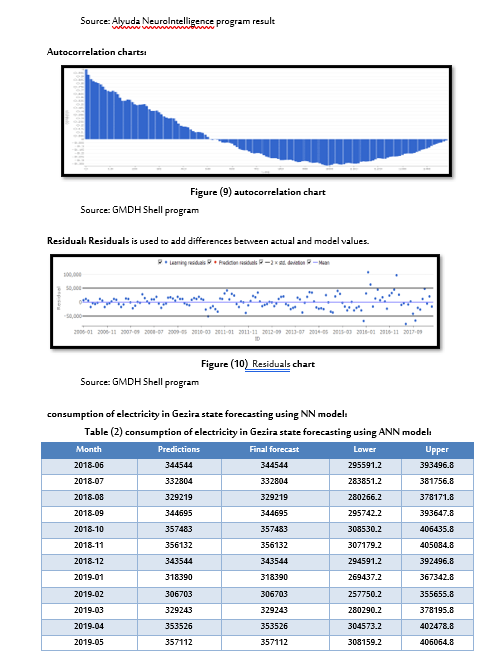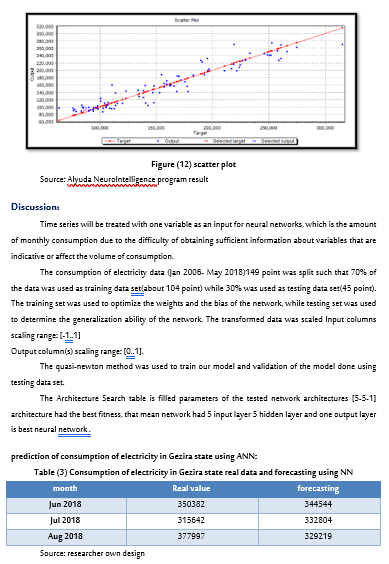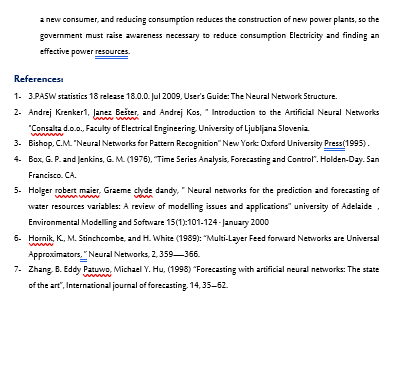==> أرسل بحثك <==